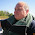How to draw Lewis dot structures | POCl3 Phosphorous oxychloride | Chemistry Net

# How to draw Lewis dot structures | POCl3 Phosphorous oxychloride

A simple procedure for writing Lewis dot structures was given in a previous post entitled “Lewis Structures and the Octet Rule”.
Several worked examples relevant to this procedure were given in previous posts please see the Sitemap - Table of Contents (Lewis Electron Dot Structures).

Let us examine the case of phosphorous oxychloride POCl3. How can we construct the POCl3 resonance Lewis dot structure?

Step 1: Connect the atoms with single bonds. The less electronegative is the phosphorous atom. Hence, the P atom is going to be the central atom. Recall that electronegativity decreases as we move away from the fluorine atom in the periodic chart.Fig.1: Connect the atoms of the POCl3 molecule with single bonds

Step 2:  Calculate the # of electrons in π bonds (multiple bonds) using  formula (1)

Where n in this case is 5. Where V = (7 +5 + 7 + 6 + 7 )  = 32 , V is the number of valence electrons of the POCl3.molecule.
Therefore, P = 6n + 2 – V = 6 * 5 + 2 – 32  = 0     So, there is no double bond.

Step 3 & 4: The  resonance electron dot structures  of POCl3 are as follows:Fig. 2 : Electron dot structures of POCl3

Please, watch the following video on how to draw Lewis structures:

1.1.